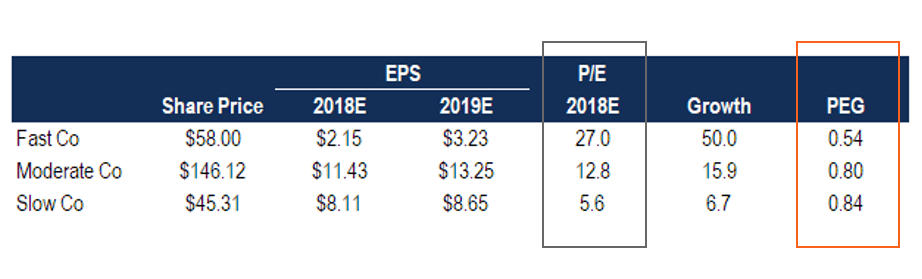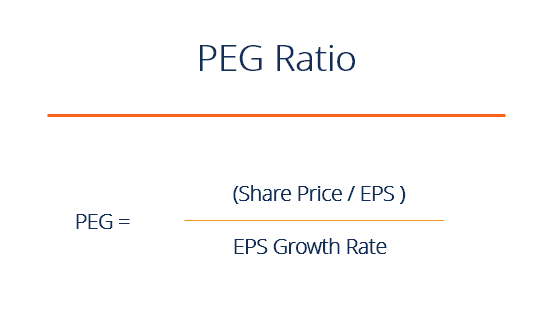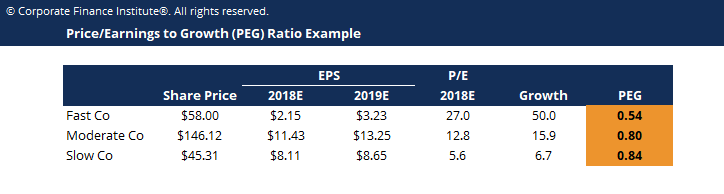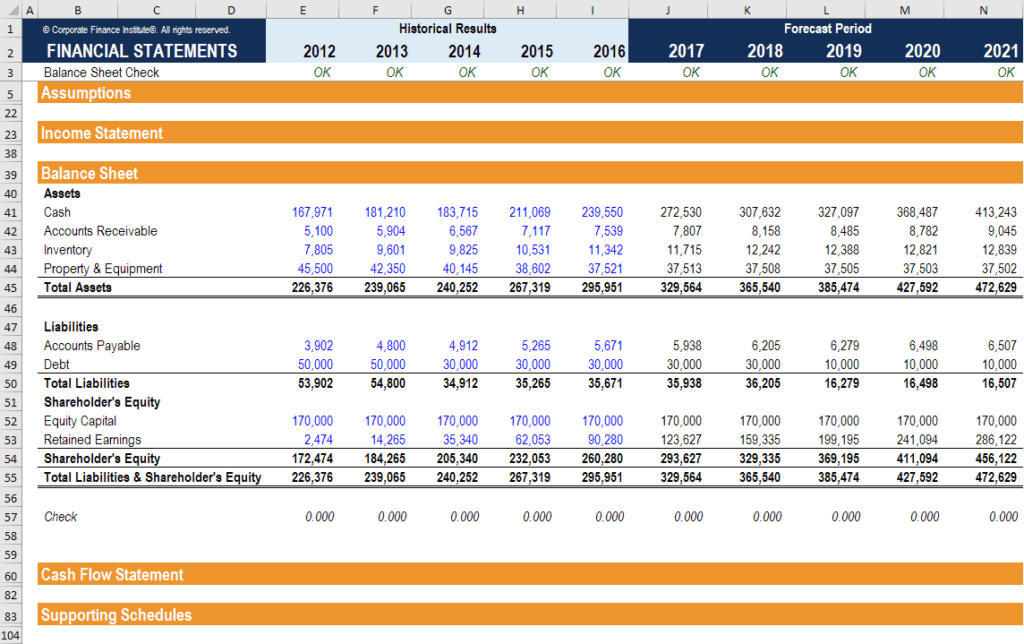# PEG Ratio

## What is the Price-Earnings-Growth PEG ratio?

The PEG ratio is a company’s Price/Earnings ratio divided by its earnings growth rate over a period of time (typically the next 1-3 years).  The PEG ratio adjusts the traditional P/E ratio by taking into account the growth rate in earnings per share that are expected in the future.  This can help “adjust” companies that have a high growth rate and a high price to earnings ratio.### What is the PEG ratio formula?

The PEG ratio formula for a company is as follows:

PEG = Share Price / Earnings per share / Earnings per Share growth rate### Example of the PEG ratio calculation

Using the example shows in the table at the top of this guide, there are three companies we can compare: Fast Co, Moderate Co, and Slow Co.

Fast Co has a price of \$58.00, 2018 EPS of \$2.15, and 2019 EPS of \$3.23

Fast Co therefore as a P/E of 27.0x, which divided by its growth rate of 50 results in a PEG ratio of 0.54

Moderate Co has a price of \$146.12, 2018 EPS of \$11.43, and 2019 EPS of \$13.25

Moderate Co has a P/E of 12.8x, divided by growth in EPS of 15.9 results in a PEG of 0.80

Slow Co has a price of \$45.31, 2018 EPS of \$8.11, and 2019 EPS of \$8.65

Slow Co’s P/E is 5.6x, divided by growth of 6.7 results in a PEG ratio of 0.84### How to interpret the PEG ratio

Based on the above examples, Fast Co has the highest P/E ratio at 27-times, and on the surface, may look expensive.  Slow Co, on the other hand, has a very low PE ratio of only 5.6-times, which may cause investors to think it’s cheap.

There is one major difference between these two companies (all else being equal), which is that Fast Co is growing it’s earnings per share at a much faster rate than Slow Co.  Given how quickly Fast Co is growing, it seems reasonable to pay more for the stock.  One way of estimating how much more is by divided each company’s PE ratio by its growth rate.  When we do this, we see that Fast Co may actually be “cheaper” than Slow Co given its increasing EPS.

### Risks of using the PEG ratio

While the ratio helps adjust for growth over a period of time, it typically only takes into account a short time period, such as 1-3 years.  For this reason, one of two years of high growth may overstate the benefit of buying the faster-growing company.  The opposite is true with Slow Co.

Additionally, the ultimate driver of value of a company Free Cash Flow, and growth in EPS may not result in growth in cash flow (i.e. high capital expenditures required to achieve the earnings growth).

While it is often a helpful adjustment to P/E, it should be considered as only one of various factors when valuing a company.

### Financial modeling to value a company

Using the PEG ratio formula can be useful, but ultimately financial modeling is the best way to account for all aspects of a company’s growth profile when performing a valuation.  Building a Discounted Cash Flow (DCF) model typically takes into account about 5 years of forecasted growth, plus a terminal value, to arrive at the net present value of the business.

There are various types of financial models, to learn more about financial modeling and valuation check out our online financial modeling courses.This has been a guide to adjusting the traditional PE ratio by dividing by a company’s growth rate in earnings per share to arrive at the PEG ratio.

• Valuation techniques
• The ultimate cash flow guide
• Forecasting an income statement
• Comparable company analysis

### Valuation Techniques

Learn the most important valuation techniques in CFI’s Business Valuation course!

Step by step instruction on how the professionals on Wall Street value a company.

Learn valuation the easy way with templates and step by step instruction!